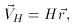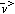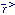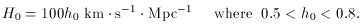B. The Expanding Universe

In the mid-twenties, Edwin Hubble was observing a group of objects known as spiral nebulae (7) These nebulae contain a very important class of stars known as Cepheid Variables. Because the Cepheids have a characteristic variation in brightness , Hubble could recognize these stars at great distances and then compare their observed luminosity to their known luminosity. This allowed him to compute the distance to the stars, since luminosity is inversely proportional to the square of the distance . The intrinsic, or absolute, luminosity is calculated from simple models that have been commensurate with observations of near Cepheids.

When Hubble compared the distance of the Cepheids to their velocities (computed by the redshift of their spectrum) he found a simple linear relationship,(1)

whereH is the velocity of the galaxy, H is the so-called Hubble Constant, andis the displacement of the galaxy from the Earth. It will be shown later that the Hubble constant is not actually a constant, but can be a function of time depending on the chosen model. The standard notation is to adopt H0 as the `current' observed Hubble parameter, whereas H = H(t) is referred to as the Hubble constant. The current accepted value of the Hubble parameter is,(2)

The unit of length, Mpc, stands for Megaparsec (8)

Hubble's interpretation of his data was crucial in helping determine the correct model for the universe. Hubble had found that the galaxies, on average, were receding away from us at a velocity proportional to their distance from us (1). This suggests a homogeneous, isotropic, and expanding universe. By this finding, the choices of cosmological models became greatly restricted.

Perhaps it is worth mentioning that the above analysis by Hubble is not quite as easily done as one might think. One factor that must be considered in the calculation of the Hubble velocity field (1) is the concept of peculiar velocity. This is the name given to the motion of a galaxy, relative to the CRF, due to its rotation and motion as influenced by the gravitational pull of nearby clusters. This speed, vp± 500 km s-1, can be neglected at far distances where the Hubble speed, VH >> 500 km s-1. Thus, when Hubble conducted his survey most of the nebulae were too near to rule out an effect by the peculiar velocity. As a result, Hubble found H0500 km s-1 Mpc, much greater than the value obtained today from surveys of type Ia supernovae (9)

7 It would later be found that most of these nebula were in fact galaxies . Back.
8 1 Mpc = 106 parsecslight years3 x 1016 meters.
A parsec is the distance to an object that has an angular parallax of 1° and a baseline of 1 A.U. For more on Observational Astronomy see . Back.
9 Supernova Ia, like Cepheid Variables, have a known `signature' and can therefore be used as `Standard Candles', but unlike the Cepheids, supernovae are much more luminous and can therefore be seen at much greater distance . Back.